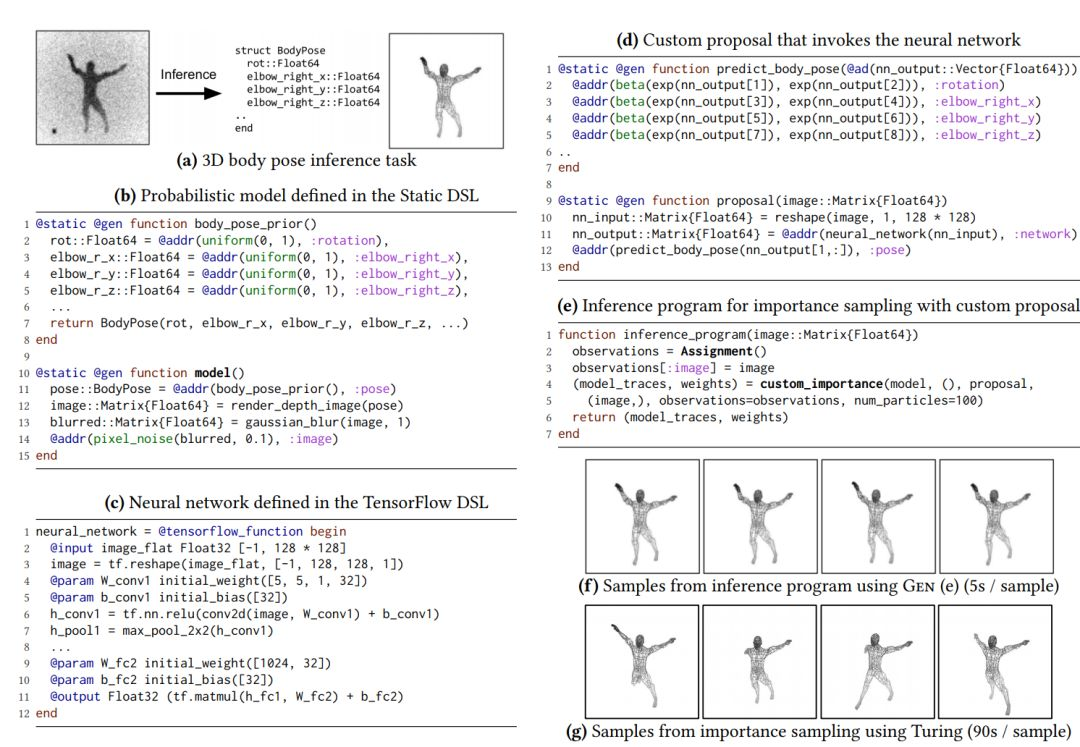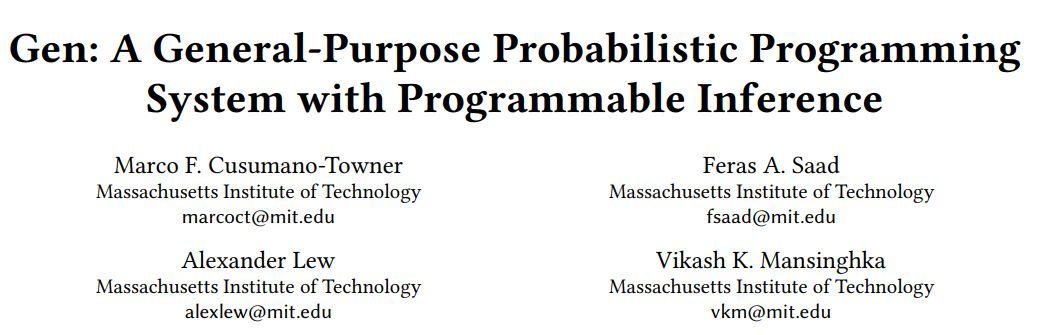### 【轉載】無需數學就能寫AI，MIT提出AI專用編程語言Gen「Gen 是第一個足以涵蓋計算機視覺和數據科學中不同案例的靈活、自動化、高效的系統，且性能非常好。」MIT 腦與認知科學系（Department of Brain and Cognitive Sciences）的研究員 Vikash K. Mansinghka 説，他負責運行概率計算項目。

2015 年，谷歌開源 TensorFlow 以來，它已經成為了最為流行的深度學習框架。然而，即使 TensorFlow 足夠自動化和高效，但它只側重於深度學習模型，對範圍更廣的人工智能而言，這些模型既昂貴又有限。

Mansinghka 表示：「藉助於 Gen，研究人員首次得以很容易地集成大量不同的 AI 技術。」

``using Gen``@gen function my_model(xs::Vector{Float64})`` slope = @trace(normal(0, 2), :slope)`` intercept = @trace(normal(0, 10), :intercept)`` for (i, x) in enumerate(xs)`` @trace(normal(slope * x + intercept, 1), "y-\$i")`` end``end``

``function my_inference_program(xs::Vector{Float64}, ys::Vector{Float64}, num_iters::Int)`` # Create a set of constraints fixing the `` # y coordinates to the observed y values`` constraints = choicemap()`` for (i, y) in enumerate(ys)`` constraints["y-\$i"] = y`` end`` `` # Run the model, constrained by `constraints`,`` # to get an initial execution trace`` (trace, _) = generate(my_model, (xs,), constraints)`` `` # Iteratively update the slope then the intercept,`` # using Gen's metropolis_hastings operator.`` for iter=1:num_iters`` (trace, _) = metropolis_hastings(trace, select(:slope))`` (trace, _) = metropolis_hastings(trace, select(:intercept))`` end`` `` # From the final trace, read out the slope and`` # the intercept.`` choices = get_choices(trace)`` return (choices[:slope], choices[:intercept])``end``

``xs = [1., 2., 3., 4., 5., 6., 7., 8., 9., 10.]``ys = [8.23, 5.87, 3.99, 2.59, 0.23, -0.66, -3.53, -6.91, -7.24, -9.90]``(slope, intercept) = my_inference_program(xs, ys, 1000)``println("slope: \$slope, intercept: \$intercept")``• 生成函數接口，一種封裝概率和/或可微計算的抽象

• 具有自定義編譯器的特定領域語言，對不同的靈活性/性能進行權衡

• 能夠編碼條件獨立性和重複計算常見模式的連結符，支持緩存加速

• 標準推理庫，支持在 Gen 中編寫作為程序的自定義建議分佈

https://hk.wxwenku.com/d/201099486